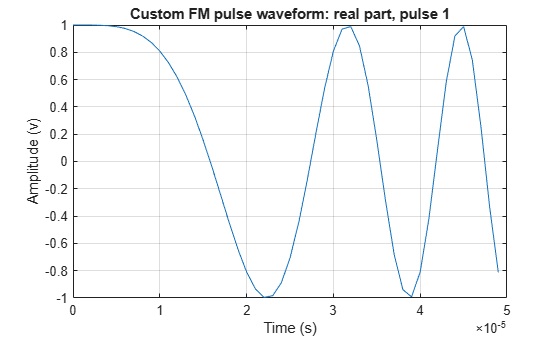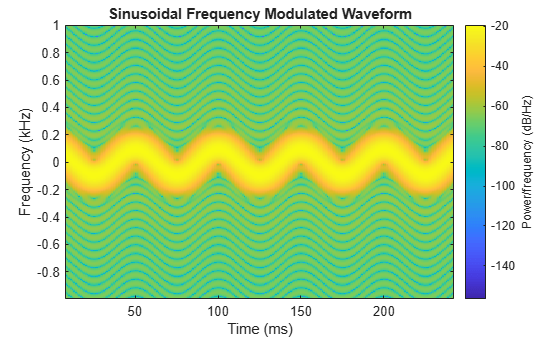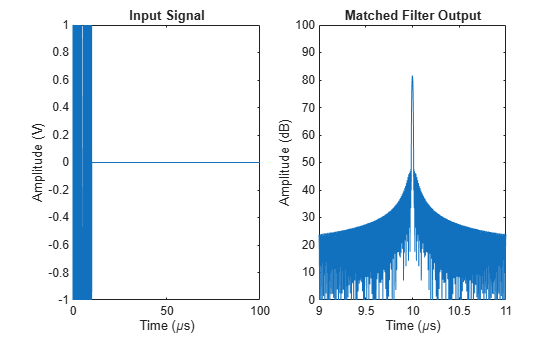# phased.CustomFMWaveform

Custom FM pulse waveform

Since R2023a

## Description

The `phased.CustomFMWaveform` System object™ lets you define a waveform with a user-defined frequency modulation (FM) and waveform envelope.

To create the waveform:

1. Create the `phased.CustomFMWaveform` object and set its properties.

2. Call the object with arguments, as if it were a function.

## Creation

### Syntax

``waveform = phased.CustomFMWaveform()``
``waveform = phased.CustomFMWaveform(Name=Value)``

### Description

example

````waveform = phased.CustomFMWaveform()` creates a custom FM pulse `waveform` System object with linear frequency modulation and a rectangular envelope.```

example

````waveform = phased.CustomFMWaveform(Name=Value)` sets additional properties using `Name`-`Value` arguments. You can specify additional name-value pair arguments in any order as (`Neme1`=`Value1`,…,`NameN`=`ValueN`).```

## Properties

expand all

Unless otherwise indicated, properties are nontunable, which means you cannot change their values after calling the object. Objects lock when you call them, and the `release` function unlocks them.

If a property is tunable, you can change its value at any time.

Signal sample rate, specified as a positive scalar. The ratio of sample rate to pulse repetition frequency must be a positive integer, so the number of samples in each pulse must be an integer value. Units are in Hertz.

Example: 100e3

Data Types: `double`

Method to set pulse duration (pulse width), specified as `'Pulse width'` or `'Duty cycle'`. This property determines how you set the pulse duration.

• When you set this property to `'Pulse width'`, set the pulse duration directly using the `PulseWidth` property.

• When you set this property to `'Duty cycle'`, set the pulse duration from the values of the `PRF` and `DutyCycle` properties. The pulse width is equal to the value of the `DutyCycle` property divided by the value of the `PRF` property.

Data Types: `char` | `string`

Pulse time duration, specified as a positive scalar. The value must satisfy `PulseWidth <= 1./PRF`. Units are in seconds.

#### Dependencies

To enable this property, set the `DurationSpecification` property to `'Pulse width'`.

Data Types: `double`

Pulse duty cycle, specified as a positive scalar in the range `[0,1]`. The pulse width is the value of the `DutyCycle` property divided by the value of the `PRF` property. This quantity is dimensionless.

Example: `0.75`

#### Dependencies

To enable this property, set the `DurationSpecification` property to `'Duty cycle'`.

Data Types: `double`

Pulse repetition frequency (PRF), specified as a scalar or a row vector. Units are in Hz. The pulse repetition interval (PRI) is the inverse of the pulse repetition frequency PRF value. The PRF must satisfy these restrictions:

• The product of `PRF` and `PulseWidth` must be less than or equal to one. This condition requires that the pulse width is less than one PRI. For the phase-coded waveform, the pulse width is the product of the values of the `ChipWidth` and `NumChips` properties.

• The ratio of `SampleRate` to `PRF` must be an integer. This condition requires that the number of samples in one PRI is an integer.

You can set the value of PRF using the `PRF` property settings alone or using property settings in conjunction with the `prfidx` input argument of the object.

• When `PRFSelectionInputPort` is `false`, you set the PRF using the `PRF` properties alone. You can:

• Implement a constant PRF by specifying the `PRF` property as a positive real-valued scalar.

• Implement a staggered PRF by specifying the `PRF` property as a row vector with positive real-valued elements. Each call to the object uses successive elements of this vector as the PRF. Once the object reaches the last element of the vector, it continues the process cyclically with the first element of the vector.

• When `PRFSelectionInputPort` is `true`, you can set the PRF value using the `PRF` property in conjunction with the `prfidx` input argument. You implement a selectable PRF by specifying the `PRF` property as a row vector with positive real-valued elements. When you execute the object, the object selects a PRF by using the index you specify in the `prfidx` input argument to index into the PRF vector.

In all cases, the number of output samples is fixed when you set the `OutputFormat` property to `'Samples'`. When you use a varying PRF and also set the `OutputFormat` property to `'Pulses'`, the number of samples can vary.

Data Types: `double`

Enable PRF selection input, specified as `false` or `true`. When you set this property to `true`, you can pass an index argument to the object to select a predefined value from the `PRF` property vector. When you set this property to `false`, the object uses the `PRF` property to define the PRF sequence used in the simulation.

Data Types: `logical`

Waveform frequency modulation, specified as a length-M real-valued vector, function handle, or cell array.

• If the `FrequencyModulation` property is a vector, it specifies samples of the instantaneous frequency at M points as [f1, f2, …, fM]. The waveform sweeps the specified frequencies such that for the kth pulse with start time tk and duration Tk, the instantaneous frequency at time

`${t}_{m}={t}_{k}+\left(m-1\right){T}_{k}/\left(M-1\right)$`

is equal to fm where tktmtk + Tk and m = 1...M. The instantaneous frequencies between time tm and tm+1 are found by linearly interpolating between fm and fm+1. The resulting custom FM waveform is a piecewise linear FM (LFM) waveform consisting of M-1 LFM sections of equal duration.

• If the `FrequencyModulation` property is a function handle, the function must have the following syntax: `f = fmFcn(t)` where `f` is the instantaneous frequency at time `t`. `t` is the time at which to compute the instantaneous frequency. The values in `t` are between 0 and the pulse width.

• If the `FrequencyModulation` property is a cell array, then the first cell must be a function handle as specified above. The remaining entries in the cell array are the additional input arguments to the function, if any.

Data Types: `double`

Waveform envelope function, specified as `'Rectangular'`, `'Gaussian'`, `'Hamming'`, `'Chebyshev'`, `'Hann'`, `'Kaiser'`, `'Taylor'`, or `'Custom'`.

When you set `Envelope` is set to `'Custom'` must use the `CustomEnvelope` property to specify a custom envelope.

Example: `'Taylor'`

Data Types: `char` | `string`

Sidelobe level for a Kaiser, Chebyshev, or Taylor window used as the waveform envelope, specified as a positive scalar. Units are in dB.

#### Dependencies

To enable this property, set the `Envelope` property to `'Kaiser'`, `'Chebyshev'`, or `'Taylor'`.

Data Types: `double`

User-defined waveform envelope, specified as a function handle or cell array.

• If `CustomEnvelope` is a function handle, the specified function uses the window length as input and generates appropriate window coefficients.

• If `CustomEnvelope` is a cell array, then the first cell must be a function handle. The specified function takes the window length as the first input argument, with other additional input arguments if necessary, and generates appropriate window coefficients. The remaining entries in the cell array serve as additional input arguments to the function.

Example: `{@chebwin,512,100}`

#### Dependencies

To enable this property, set the `Envelope` property to `'Custom'`.

Data Types: `cell` | `function_handle`

Source of frequency offset, specified as `'Property'` or `'Input port'`.

• When you set this property to `'Property'`, the frequency offset is determined by the value of the `FrequencyOffset` property.

• When you set this property to `'Input port'`, the frequency offset is determined by the input argument `freqoffset` when calling the object.

Example: `'Input port'`

Data Types: `char` | `string`

Frequency offset, specified as a scalar. Units are in Hz.

Example: `150.0`

#### Dependencies

To enable this property, set the `FrequencyOffsetSource` property to `'Property'`.

Data Types: `double`

Format of output signal, specified as `'Pulses'` or `'Samples'`.

• When you set the `OutputFormat` property to `'Pulses'`, the output of the object takes the form of multiple pulses specified by the value of the `NumPulses` property. The number of samples per pulse can vary if you change the PRF during the simulation.

• When you set the `OutputFormat` property to `'Samples'`, the output of the object takes the form of multiple samples. In this case, the number of output signal samples is the value of the `NumSamples` property and is fixed.

Data Types: `char` | `string`

Number of samples in each output of the object, specified as a positive integer.

#### Dependencies

To enable this property, set the `OutputFormat` property to `'Samples'`.

Data Types: `double`

Number of pulses in each output, specified as a positive integer.

#### Dependencies

To enable this property, set the `OutputFormat` property to `'Pulses'`.

Data Types: `double`

Enable PRF output, specified as `false` or `true`. Set this property to `true` to output the PRF.

#### Dependencies

To enable this property, set the `OutputFormat` property to `'Pulses'`.

Data Types: `logical`

Enable matched filter coefficients output, specified as `false` or `true`. Set this property to `true` to enable the object the output of the matched filter coefficients of the waveform used during the simulation.

Data Types: `logical`

## Usage

### Syntax

``Y = waveform()``
``Y = waveform(prfidx)``
``Y = waveform(freqoffset)``
``[Y,PRF] = waveform(___)``
``[Y,coeff]= waveform()``

### Description

````Y = waveform()` returns samples of the custom nonlinear FM pulse in a column vector `Y`. `Y` can contain a certain number of pulses or a certain number of samples.```
````Y = waveform(prfidx)` specifies the index of the PRF vector, `prfidx`. The object uses the index is used to identify the entries specified in the PRF property. To enable this syntax, set the `PRFSelectionInputPort` property to `true`.Use this syntax for the cases where you need to dynamically select the transmitted pulse. In such situations, the `PRF` property includes a list of predetermined PRF values. During the simulation, based on PRF index input, the object selects one of the PRFs values for the PRF for the next transmission.The transmission always finishes the current pulse before starting the next pulse. Therefore, when you set the `OutputFormat` property to `'Samples' ` and then specify the `NumSamples` property to be shorter than a pulse, the object can ignore the PRF index during a given simulation step if needs the entire output to finish the previously transmitted pulse.```
````Y = waveform(freqoffset)` specifies the value of the frequency offset `freqoffset`. The offset generates the waveform with a frequency offset . Use this syntax when you need to update the transmit pulse frequency dynamically. To enable this syntax, set the `FrequencyOffsetSource` property to `'Input port'`. ```
````[Y,PRF] = waveform(___)` also returns the current `PRF`. To enable this syntax, set the `PRFOutputPort` property to `true` and set the `OutputFormat` property to `'Pulses'`.```
````[Y,coeff]= waveform()` returns the matched filter coefficients `coeff` when you set the `CoefficientsOutputPort` property to `true`.```

You can combine optional input and output arguments when you set their enabling properties are set. List optional inputs and outputs in the same order as the order of the enabling properties. For example,

`[Y,PRF,coeff] = waveform(prfidx,freqoffset)`

### Input Arguments

expand all

Index of PRF, specified as a positive integer. The index identifies the entries in the `PRF` property. To enable this syntax, set the `PRFSelectionInputPort` property to `true`. Use this syntax when you need to dynamically select the transmit pulse. In such situations, the PRF property includes a list of predetermined values. During the simulation, based on the PRF index input, the object selects one of the PRFs is selected as the PRF for the next transmission.

Frequency offset, specified as a finite scalar. The object generates the waveform with a frequency offset. Use this syntax when you need to update the transmit pulse frequency dynamically.

#### Dependencies

To use this argument, set the `FrequencyOffsetSource` property to `'Input port'`.

Data Types: `double`

### Output Arguments

expand all

Pulse repetition frequency, returned as a scalar. When you set the `PRFOutputPort` property to true it returns the current PRF used by the system. Units are in Hz.

#### Dependencies

To enable this argument, set the `PRFOutputPort` property to `true` and set the `OutputFormat` to `'Pulses'`.

Matched filter coefficients, returned as an NZ-by-1 complex-valued vector or an NZ-by-M complex-valued matrix.

• If you set `OutputFormat` to `'Pulses'` and `NumPulses` is 1, the object returns `coeff` as an NZ-length vector. NZ corresponds to the pulse width.

• If you set `OutputFormat` to `'Pulses'` with `NumPulses` greater than 1 or `OutputFormat` is `'Samples'` and `DurationSpecification` is ```'Pulse width'```, `coeff` is returned as an NZ-length vector. NZ corresponds to the pulse width.

• If `OutputFormat` is set to `'Pulses'` with `NumPulses` greater than 1 or `OutputFormat` is set to `'Samples'` and `DurationSpecification` is set to `'Duty cycle'` with only one unique PRF value, `coeff` is returned as an NZ-length vector. NZ corresponds to the pulse width.

• If `OutputFormat` is `'Pulses'` with `NumPulses` greater than 1 or `OutputFormat` is `'Samples'` and `DurationSpecification` is ```'Duty cycle'```, `coeff` is returned as an NZ-by-M matrix. NZ corresponds to the maximum pulse width and M corresponds to the number of unique PRFs.

#### Dependencies

To enable this argument, set the `CoefficientsOutputPort` property to `true`.

Data Types: `double`
Complex Number Support: Yes

## Object Functions

To use an object function, specify the System object as the first input argument. For example, to release system resources of a System object named `obj`, use this syntax:

`release(obj)`

expand all

 `bandwidth` Waveform bandwidth `getMatchedFilter` Matched filter coefficients derived from waveform `plot` Plot pulse waveform
 `step` Run System object algorithm `release` Release resources and allow changes to System object property values and input characteristics `reset` Reset internal states of System object

## Examples

collapse all

Create a custom FM waveform with the default frequency modulation and envelope function.

`waveform = phased.CustomFMWaveform() `
```waveform = phased.CustomFMWaveform with properties: SampleRate: 1000000 DurationSpecification: 'Pulse width' PulseWidth: 5.0000e-05 PRF: 10000 PRFSelectionInputPort: false FrequencyModulation: [0 100000] Envelope: 'Rectangular' FrequencyOffsetSource: 'Property' FrequencyOffset: 0 OutputFormat: 'Pulses' NumPulses: 1 PRFOutputPort: false CoefficientsOutputPort: false ```

Display the real part of the waveform.

`plot(waveform)`Create a sinusoidal frequency modulated waveform with a bandwidth of 200 Hz, a pulse width of 0.25 s, and a modulation frequency of 20 Hz.

```BW = 200; T = 0.25; fm = 20; fs = 10*BW; freqfunc = @(t)(BW/2)*cos(2*pi*fm*t); waveform = phased.CustomFMWaveform(SampleRate=fs, ... PulseWidth=T,FrequencyModulation=freqfunc,PRF=1/T); wav = waveform();```

Display the spectrogram of the waveform.

```spectrogram(wav,32,30,512,fs,'yaxis','centered') title('Sinusoidal Frequency Modulated Waveform')```Create a nonlinear FM waveform derived from a power spectral density function shaped as a Taylor window with -35 dB sidelobes. The pulse bandwidth is 120 MHz and the pulse duration is$10\mu$sec. Generate matched filter coefficients and then apply a matched filter. Plot the resulting matched filter output to display the range sidelobe levels.

```BW = 120e6; T = 10e-6; fs = 10*BW;```

Generate 200 points of a waveform with instantaneous frequency values defined by a Taylor window. The window has -35 dB sidelobe levels.

```w = taylorwin(200,4,-35); freq = nlfmspec2freq(BW,w); waveform = phased.CustomFMWaveform('SampleRate',fs, ... 'PulseWidth',T,'FrequencyModulation',freq, ... 'OutputFormat','Pulses','CoefficientsOutputPort',true); disp(['Bandwidth = ',num2str(bandwidth(waveform)/1e6),' MHz'])```
```Bandwidth = 119.9644 MHz ```

Obtain the matched filter coefficients from the waveform.

```[wav,coeff] = waveform(); filter = phased.MatchedFilter('CoefficientsSource','Input port'); mfout = filter(wav,coeff);```

Plot input signal and matched filter output.

```t = (0:numel(wav)-1)/fs; figure subplot(121) plot(t*1e6,real(wav)) xlabel('Time (\mus)') ylabel('Amplitude (V)') title('Input Signal') subplot(122) plot(t*1e6,mag2db(abs(mfout))) xlabel('Time (\mus)') ylabel('Amplitude (dB)') title('Matched Filter Output') xlim([9 11]) ylim([0 100])```Collins, T., and P. Atkins. "Nonlinear frequency modulation chirps for active sonar." IEE Proceedings-Radar, Sonar and Navigation 146.6 (1999): 312-316.

 Levanon, Nadav, and Eli Mozeson. Radar signals. John Wiley & Sons, 2004, pp. 92-93.

 Doerry, Armin Walter. "Generating nonlinear FM chirp waveforms for radar". No. SAND2006-5856. Sandia National Laboratories (SNL), Albuquerque, NM, and Livermore, CA (United States), 2006.

 Cook, C. E. "A class of nonlinear FM pulse compression signals." Proceedings of the IEEE 52.11 (1964): 1369-1371.

 Yang, J., and T. K. Sarkar. "Doppler‐invariant property of hyperbolic frequency modulated waveforms." Microwave and optical technology letters 48.6 (2006): 1174-1179.

 Melvin, William L., and James Scheer. Principles of modern radar: advanced techniques. SciTech Pub., 2013.

 Alphonse, Sebastian, and Geoffrey A. Williamson. "Evaluation of a class of NLFM radar signals." EURASIP Journal on Advances in Signal Processing 2019.1 (2019): 1-12.